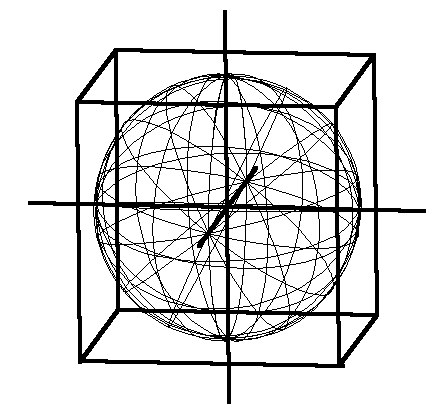Cube Root

In mathematics, a cube root of a number, denoted x1/3, is a number a such that a3 = x. For example, the real cube root of 8 is 2, because 23 = 8.

The x, y, or z side or edge of a cube. Also diameter of a sphere enclosed and coincident to a circumscribing cube.Figure 6.18 - Sphere Circumscribed by Cube (click to enlarge)